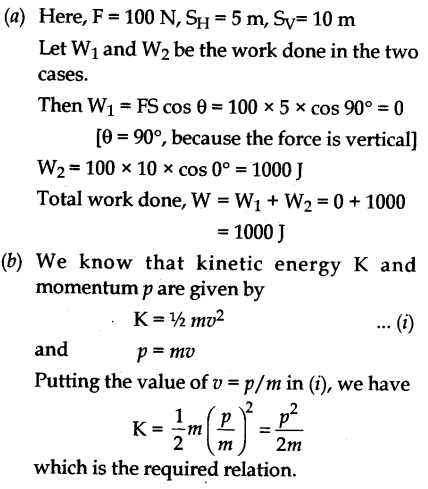# (a) A person is holding a bucket of water by applying a vertical force of 100 N. He first moves a horizontal distance of 5 m and then climbs up through stairs a vertical distance of 10 m. Calculate the total work done the person on the bucket

(a) A person is holding a bucket of water by applying a vertical force of 100 N. He first moves a horizontal distance of 5 m and then climbs up through stairs a vertical distance of 10 m. Calculate the total work done the person on the bucket.
(b) Establish the relationship between kinetic energy and momentum of a body.